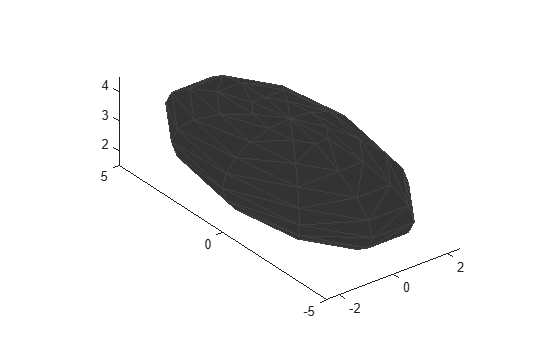# scaleToFit

Auto-scale object mesh to match specified cuboid dimensions

## Syntax

``scaledMesh = scaleToFit(mesh,dims)``

## Description

example

````scaledMesh = scaleToFit(mesh,dims)` auto-scales the object mesh to match the dimensions of a cuboid specified in the structure `dims`.```

## Examples

collapse all

Create an `extendedObjectMesh` object and auto-scale the object to the required dimensions.

Construct a sphere mesh of unit dimensions.

`sph = extendedObjectMesh('sphere');`

Auto-scale the mesh to the dimensions in `dims`.

```dims = struct('Length',5,'Width',10,'Height',3,'OriginOffset',[0 0 -3]); sph = scaleToFit(sph,dims);```

Visualize the mesh.

`show(sph);`## Input Arguments

collapse all

Extended object mesh, specified as an `extendedObjectMesh`

Dimensions of the cuboid to scale an object mesh, specified as a `struct` with these fields:

• `Length` – Length of the cuboid

• `Width` – Width of the cuboid

• `Height` – Height of the cuboid

• `OriginOffset` – Origin offset in 3-D coordinates

All the dimensions are in meters.

Data Types: `struct`

## Output Arguments

collapse all

Scaled object mesh, returned as an `extendedObjectMesh` object.

### Functions

Introduced in R2020a